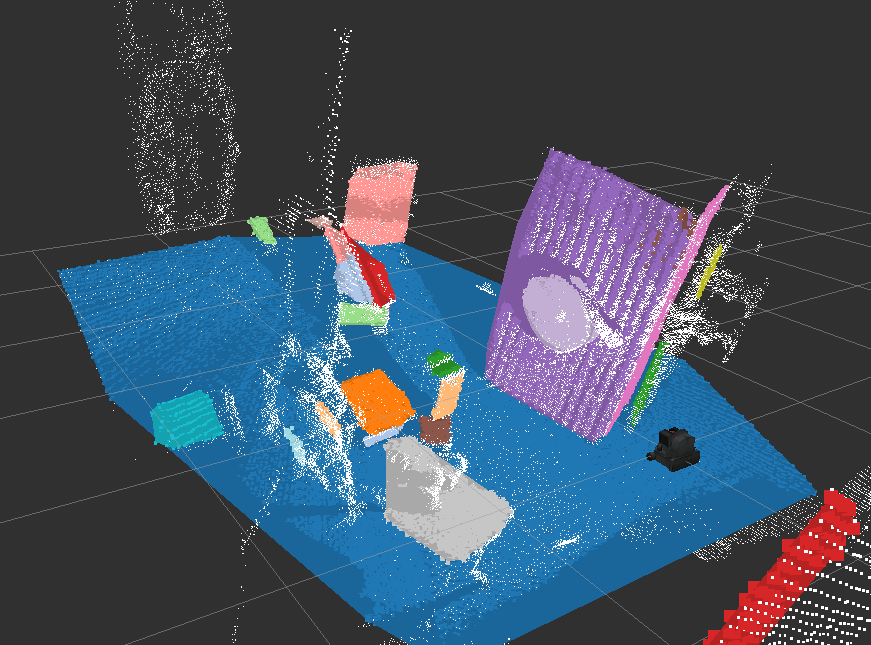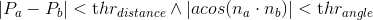# RegionGrowingMultiplePlaneSegmentation¶jsk_pcl/RegionGrowingMultiplePlaneSegmentation

jsk_pcl/RegionGrowingMultiplePlaneSegmentation estimates multiple planes from pointcloud.

It extracts planes based on region growing and evaluation function of connectivity if based on the following equation:## Subscribing Topics¶

• ~input (sensor_msgs/PointCloud2):

input pointcloud.

Point type should be pcl::PointXYZRGB.

• ~input_normal (sensor_msgs/PointCloud2):

normal pointcloud of ~input.

Point type should be pcl::Normal.

## Publishing Topics¶

• ~output/clustering_result (jsk_recognition_msgs/ClusterPointIndices):

Result of region growing as cluster.

• ~output/inliers (jsk_recognition_msgs/ClusterPointIndices):

Set of indices of the polygons.

• ~output/coefficients (jsk_recognition_msgs/ModelCoefficientsArray):

Array of coefficients of the polygons.

• ~output/polygons (jsk_recognition_msgs/PolygonArray):

Plane polygons.

• ~output/latest_time (std_msgs/Float32)

latest computation time

• ~output/average_time (std_msgs/Float32)

average computation time

## Parameters¶

• ~angular_threshold (Double, default: 0.04)

Angular threshold in [rad] to connect two points in one cluster.

• ~distance_threshold (Double, default: 0.01)

Distance threshold in [m] to connect two points in one cluster.

• ~max_curvature (Double, default: 0.1)

Before extracting planes, filtering out the points which have higer curvature than this value.

• ~min_size (Integer, default: 100)

The minimum number of the points of each plane.

• ~max_size (Integer, default: 25000)

The maximum number of the points of each plane.

Currently this parameter is disabled and unlimited is set instead.

• ~min_area (Double, default: 0.1)

The minimum area of the convex planes in [m^2].

• ~max_area (Double, default: 100)

The maximum area of the convex planes in [m^2].

• ~cluster_tolerance (Double, default: 0.1)

The spatial tolerance in [m] for new cluster candidates.

• ~ransac_refine_outlier_distance_threshold (Double, default: 0.1)

Outlier threshold in [m] for plane estimation using RANSAC.

• ~ransac_refine_max_iterations (Integer, default: 100)

The maximum number of the iterations for plane estimation using RANSAC.

Note that these parameters can be changed by dynamic_reconfigure.

## Sample¶

roslaunch jsk_pcl_ros sample_region_growing_multiple_plane_segmentation.launch